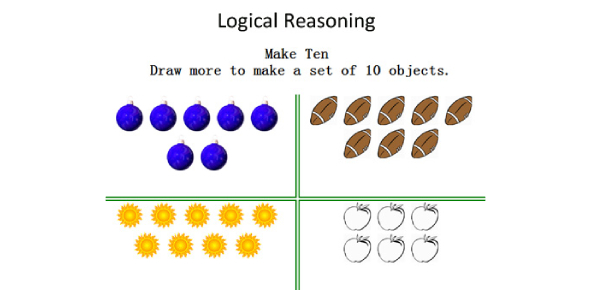# Logical Reasoning MCQ Test: Trivia

27 Questions | Total Attempts: 2108SettingsAre you up for this Logical Reasoning MCQ test? A person who is said to think logically can look deep into a situation before they agree on a course of action. Do you see yourself as having the makeup of a logical thinker? This quiz will help to test out just how true that is by giving you some questions to get you thinking. All the best and share the quiz with others to see how they think.

• 1.
What is the missing letter in this series:   a     a     b     b     ?     c
• A.

A

• B.

B

• C.

C

• D.

D

• E.

E

• 2.
What is the missing letter in this series:   c     c     d     ?     e     f     g     g     h
• A.

B

• B.

C

• C.

D

• D.

E

• E.

F

• 3.
What is the missing letter in this series:  a     z     b     ?     c     x
• A.

B

• B.

C

• C.

W

• D.

X

• E.

Y

• 4.
What is the missing letter in this series:  f     g     e     h     d     i     c     ?
• A.

A

• B.

B

• C.

J

• D.

K

• E.

I

• 5.
What is the missing letter in this series:  r     q     p     r     q     p     ?
• A.

O

• B.

P

• C.

Q

• D.

R

• E.

S

• 6.
What is the missing letter in this series:  b     e     h     k     n     ?     t
• A.

O

• B.

P

• C.

Q

• D.

R

• E.

S

• 7.
What is the missing letter in this series:  y     e     w     g     u     i     ?
• A.

S

• B.

T

• C.

R

• D.

K

• E.

I

• 8.
What is the missing letter in this series:  x     ?     p     l     h
• A.

R

• B.

S

• C.

Q

• D.

O

• E.

T

• 9.
What is the missing letter in this series:  c     c     d     f     i     ?
• A.

J

• B.

K

• C.

I

• D.

M

• E.

N

• 10.
What is the missing letter in this series:  j     g     d     k     ?     e     l
• A.

H

• B.

F

• C.

I

• D.

M

• E.

G

• 11.
What is the missing letter in this series:  a     b     d     h     ?
• A.

L

• B.

M

• C.

N

• D.

O

• E.

P

• 12.
What is the missing letter in this series:  b     g     d     i     ?     k     h
• A.

J

• B.

E

• C.

C

• D.

F

• E.

G

• 13.
What is the missing letter in this series:  a     d     i     ?
• A.

P

• B.

N

• C.

O

• D.

L

• E.

M

• 14.
What is the missing letter in this series:  g     ?     d     i     j     d     k     l     d
• A.

F

• B.

E

• C.

C

• D.

H

• E.

D

• 15.
What is the missing letter in this series:  p     q     q     ?     s     s     t     u
• A.

R

• B.

T

• C.

S

• D.

U

• E.

Q

• 16.
What is the missing letter in this series:  g     g     k     k     o     o     ?
• A.

Q

• B.

S

• C.

R

• D.

O

• E.

T

• 17.
What is the missing letter in this series:  y     e     u     i     q     m     ?
• A.

M

• B.

N

• C.

J

• D.

K

• E.

O

• 18.
What is the missing letter in this series:  v     s     p     w     t     q     ?
• A.

R

• B.

U

• C.

X

• D.

V

• E.

Y

• 19.
What is the missing letter in this series:  a     b     k     c     d     k     e     ?
• A.

F

• B.

G

• C.

K

• D.

E

• E.

H

• 20.
What is the missing letter in this series:  q     r     k     s     t     i     u     v      ?
• A.

V

• B.

Y

• C.

H

• D.

X

• E.

G

• 21.
What is the missing letter in this series:  c     j     q     x     e     ?     s
• A.

J

• B.

L

• C.

N

• D.

G

• E.

P

• 22.
What is the missing letter in this series:  y     d     j     w     f     l     u     h     ?
• A.

M

• B.

O

• C.

N

• D.

S

• E.

J

• 23.
What is the missing letter in this series:  m     o     n     n     o     m     p     ?
• A.

L

• B.

P

• C.

M

• D.

N

• E.

O

• 24.
What is the missing letter in this series:  c     d     c     d     d     d     e      d      e     ?
• A.

D

• B.

E

• C.

F

• D.

G

• E.

H

• 25.
What is the missing letter in this series:  a     b     b     d     c     f     d     ?
• A.

E

• B.

F

• C.

G

• D.

H

• E.

I

Related TopicsBack to top